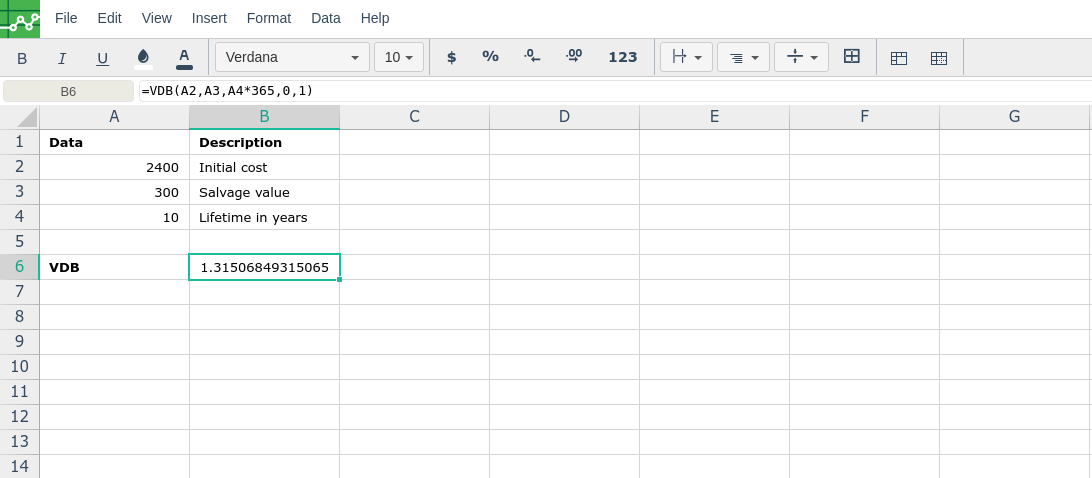# VDB

### Description

Returns the depreciation of an asset for any period you specify, including partial periods, using the double-declining balance method or some other method you specify. VDB stands for variable declining balance.

### Syntax

VDB(cost, salvage, life, start_period, end_period, [factor], [no_switch])

The VDB function syntax has the following arguments:

• Cost    Required. The initial cost of the asset.

• Salvage    Required. The value at the end of the depreciation (sometimes called the salvage value of the asset). This value can be 0.

• Life    Required. The number of periods over which the asset is depreciated (sometimes called the useful life of the asset).

• Start_period    Required. The starting period for which you want to calculate the depreciation. Start_period must use the same units as life.

• End_period    Required. The ending period for which you want to calculate the depreciation. End_period must use the same units as life.

• Factor    Optional. The rate at which the balance declines. If factor is omitted, it is assumed to be 2 (the double-declining balance method). Change factor if you do not want to use the double-declining balance method. For a description of the double-declining balance method, see DDB.

• No_switch    Optional. A logical value specifying whether to switch to straight-line depreciation when depreciation is greater than the declining balance calculation.

• If no_switch is TRUE, Microsoft Excel does not switch to straight-line depreciation even when the depreciation is greater than the declining balance calculation.

• If no_switch is FALSE or omitted, Excel switches to straight-line depreciation when depreciation is greater than the declining balance calculation.

###Error.

User does not have sufficient privileges to access this Content